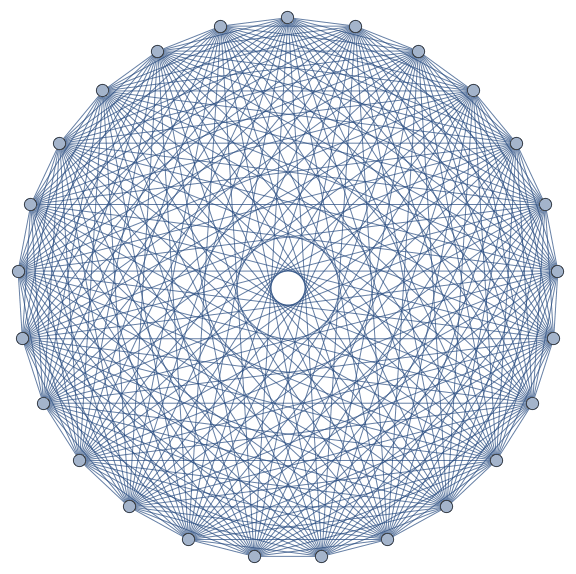#Wolfram Summer School

# Alumni# Thomas Wood

Summer School

Class of 2015

## Bio

Tommy graduated in 2013 from Queen Mary University of London with a first class master’s in theoretical physics. Since then he has been working as both a math teaching assistant and a higher education private tutor on subjects such as linear algebra, combinatorics, theory of computation, and group theory. His key interests were focused around discrete representation of space-time using computer implementation. During his final year at university, he developed a Dynamical Non-Deterministic Cellular Automata. The key was to find emergent behavior that showed some resemblance of modern physical theories of space-time and matter. This project is ongoing, and Tommy is currently attending the Wolfram Summer School to improve the efficiency of his implementations and to represent his system in greater than one dimension, where he hopes to find some interesting behavior.

## Project: Fully Connected Stochastic Substitutions (FCSS) Systems in Non-Euclidean Geometries

The specific system that will be investigated in this research project is structured much like a complete weighted graph on n vertices. Each edge, named interaction edge, consists of a string of indivisible unit parts, named space elements. The amount of these space elements effectively constitutes the weight of the corresponding edge and gives a measure of some ideal Euclidean distance between vertices. Each interaction edge evolves much like a stochastic substitution system, with each space element performing one of two actions, either duplicating (into two), or reducing (into zero), each with a corresponding probability. Furthermore, each point particle has the potential to split (into two, with one space element between them), or to merge, (the interaction edge completely reduces, resulting in one point particle). As well as these rules, each interaction edge also evolves via an action propagation mechanism, where the information of each action propagates through the system at a a rate of one space element per time-step, and does not update the state of the system until the information reaches the end of the interaction edge.

When generalizing this system to higher dimensions, the size of the weighted edges violates the constraints of flat Euclidean space. This not only implies intrinsic curvature of the system, when implemented in I dimensions, but also implies that there is one or more coordinate configuration in flat space that minimizes the stress function of the system (a measure of the configurations in different dimensions, given different distributions of weighted graphs), and we will use Mathematica’s built-in function Minimize[] to find the “best fit” for these systems while using a Euclidean fitting algorithm to find other potential satisfying configurations. The evolution of the one-dimensional interaction edge system will be explored and used to determine its behavior in higher-dimensional statistical density distributions. The effect of splitting on the system will also be investigated, as well as that of the stress minimization fitting function.## Wolfram Summer School | Champaign, IL, USA | July 3 29–July 22, 2022

APPLY NOW
• 21st Annual Wolfram Summer School
• Bentley University, Waltham, MA, USA
• June 25–July 15, 2023
• Apply now